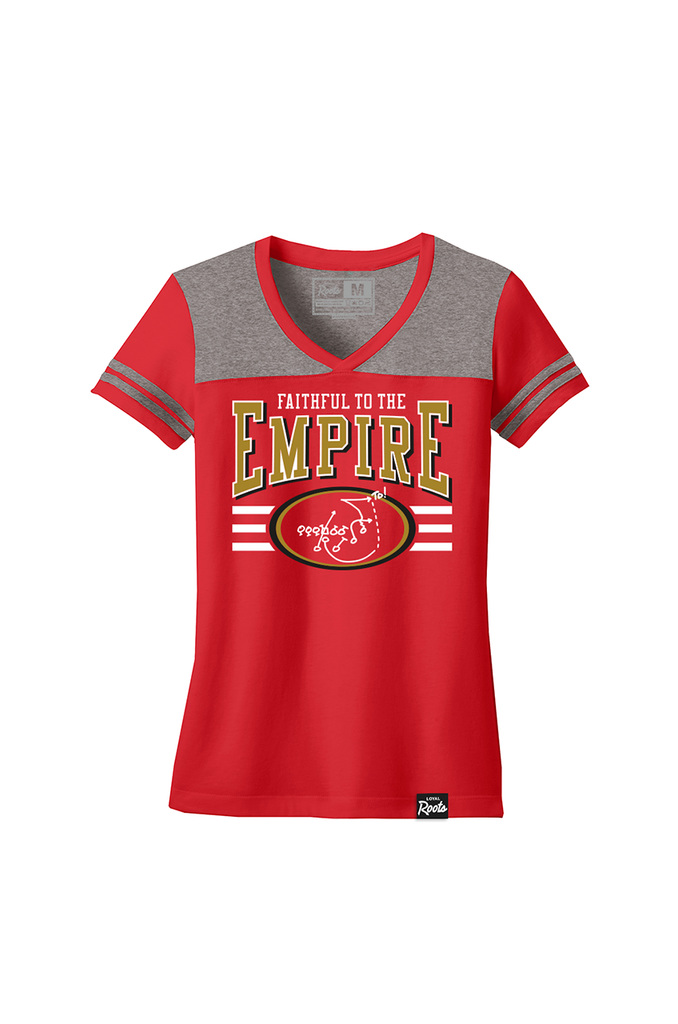Faithful to the Empire (Varsity V-Neck)

Availability: Out of stock
•\$13.00 \$26.00
4.8 oz 50/50 cotton/poly. Form-fitting. Runs small, order one size up.
Paying homage to the play that started a dynasty and built an empire! "Faithful to the Empire" features black, white and metallic gold print, custom woven label and screen printed tag.

Free Shipping

on U.S. orders over \$50

Our way of saying thanks!

Order Return

Returns within 5 days

See ‘Returns’ for details.

Measured across the chest (1” below armholes when laid flat).
 V-Neck (Relaxed) S=15” M=16.5” L=18” XL=20” 2XL=22” V-Neck/Tank/T-Shirt (Fitted) S=16.5” M=17.5” L=18.5” XL=20” 2XL=21” V-Neck/Tank/T-Shirt/Raglan (Form-Fitting) S=15.5” M=16.5” L=17.5” XL=18.5” 2XL=20” Varsity V-Neck (Form-Fitting) S=15.25” M=16.25” L=17.25” XL=18.25” 2XL=19.75” 3/4 Sleeve (Loose) S=18” M=19” L=20” XL=21” Crop Top (Relaxed) S=17.75” M=18.75” L=19.75”
Measured across the chest (1” below armholes when laid flat)
 T-Shirt S=18” M=20” L=22” XL=24” 2XL=26” 3XL=28” 4XL=30” Tank S=18” M=20” L=22” XL=24” 2XL=26” 3XL=28” Raglan S=18” M=20” L=22” XL=24” 2XL=26” 3XL=28” Longsleeve S=18” M=20” L=22” XL=24” 2XL=26” 3XL=28” Hoodie S=18” M=20” L=22” XL=24” 2XL=26” 3XL=28” Crewneck S=20” M=22” L=24” XL=26” 2XL=28” 3XL=30”